﻿

### On the nilpotent conjugacy class graph of groups

#### Abstract

The nilpotent conjugacy class graph (or NCC-graph) of a group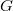$G$ is a graph whose vertices are the nontrivial conjugacy classes of$G$ such that two distinct vertices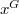$x^G$ and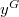$y^G$ are adjacent if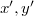$\gen{x',y'}$ is nilpotent for some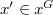$x'\in x^G$ and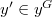$y'\in y^G$. We discuss on the number of connected components as well as diameter of connected components of these graphs. Also, we consider the induced subgraph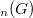$\G_n(G)$ of the NCC-graph with vertices set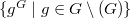$\{g^G\mid g\in G\setminus\Nil(G)\}$, where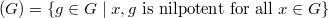$\Nil(G)=\{g\in G\mid\gen{x,g}\text{ is nilpotent for all }x\in G\}$, and classify all finite non-nilpotent group$G$ with empty and triangle-free NCC-graphs.

DOI Code: 10.1285/i15900932v37n2p77

Keywords: Triangle-free; conjugacy class; non-nilpotent group; graph

Full Text: PDF